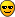Fascinating Method for Finding Pi

pi - 2 = 1/1 + 1/3 - 1/6 - 1/10 + 1/15 + 1/21 - ...

Notice the two positive terms followed by two negatives, etc., something rather unusual...and "triangular" numbers are those which are generated by n(n+1) /2...

His proof goes soemthing like this...let A equal the sum of the odd terms and B be the sum of the even terms, that is:

A = 1/1 - 1/6 + 1/15 - ...
B = 1/3 - 1/10 + 1/21 - ...

Now A = 2/(1 * 2) - 2/(3 * 4) + 2/(5 * 6) - 2/(7 *+ ...

which is also equal to

A = (2/1 - 2/2) - (2/3 - 2/4) + (2/5 - 2/6) - (2/7 - 2/+ ...

Now if we unite the terms with even denominators, he gets

- 1/1 + 1/2 - 1/3 + 1/4 - 1/5 + 1/7 - ...

and that is equal to (- log 2) according to the well-known expansion

log (1 + x) = x - x^2/2 + x^3/3 - x^4/4 + x^5/5 - ....

The terms with the odd denominators is

2/1 - 2/3 + 2/5 - 2/7 + 2/9 - 2/11 + ...

that is equal to pi/2, according to a well-known expansion for pi...

therefore A = pi/2 - log 2.

Okay, now with B...here

B = 2/(2 * 3) - 2/(4 * 5) + 2/(6 * 7) - 2/(8 * 9) + ...

that is equal to

B = (2/2 - 2/3) - (2/4 - 2/5) + (2/6 - 2/7) - (2/8 - 2/9) + ...

If we unite the terms with even denominators we get

1/1 - 1/2 + 1/3 - 1/4 + 1/5 - 1/6 + 1/7 - ...

that he is equal to log 2.

The terms with odd denominators are equal to

- 2/3 + 2/5 - 2/7 + 2/9 - 2/11 + 2/13 - 2/15 + ...

that he is equal to pi/2 - 2

So therefore B = pi/2 - 2 + log .

Now let's put it together:

If we unite the two parts A and Bm we get

A + B = pi/2 - log 2 + pi/2 - 2 + log 2 = pi - 2.

Pretty neat, huh?